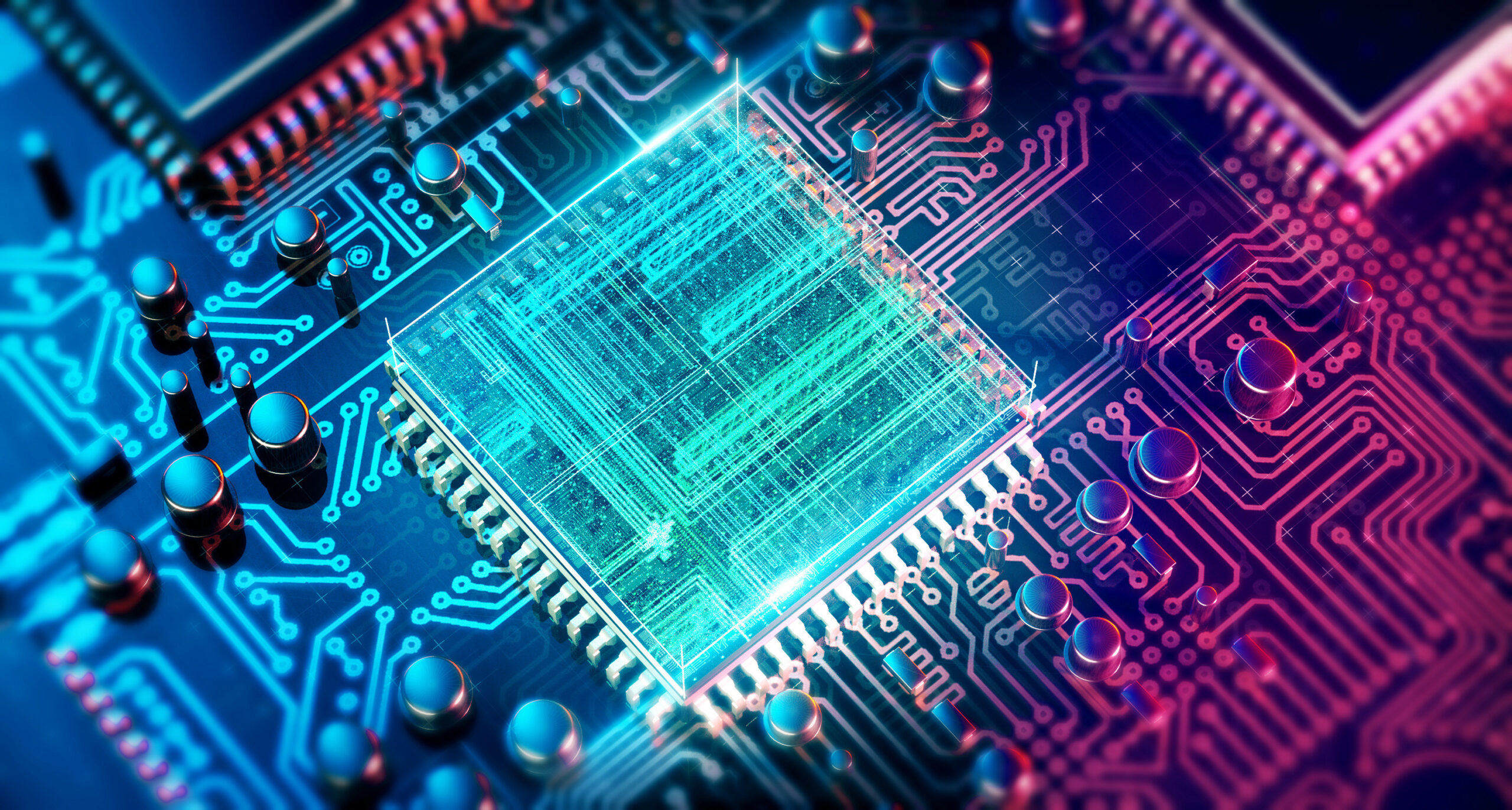## HOW QUANTUM COMPUTERS WORK?

In the classical computation model, the computer has access to memory which can be found in one of a finite set of possible states, each of which is physically distinct. The state of this memory is generally represented as a string of symbols 0 and 1. In the classical computation model, the fundamental unit of memory is called bit and the size of the memory can be measured in terms of the number of bits required to represent fully the state of the memory.  If the memory obeys laws of quantum physics, the state of memory could be found in a superposition of the possible bit strings and these strings could be found entangled with other bit strings. In the quantum computation model, the fundamental unit of memory is called a qubit which gives quantum computers its superior computing powers. In classical computers, two bits represent only two bits of information, however, in quantum computers, two qubits represent four bits of information. In quantum computers, N qubits represent 2N classical bits of information, whereas, in classical computers, N bits represent only N bits of information. Therefore, quantum computers could arrive at a result more efficiently than classical computers.